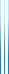# PublicationsManuscripts
Andreas Blass explained how the type-theoretic identity $T=1+T^2$ for binary trees leads to a “finitistic” identity $T^7=T$ (the solution to the former equation is a primitive sixth root of unity). More generally, he proved that two polynomials in $T$ are “equal” (under his finitistic interpretation) if and only if they agree on a primitive sixth root of unity and in terms of cardinalities. We give an exposition of this result (using work by Fiore and Leinster), using a more intuitive definition of “strong” equality, as equality given by an algorithm which also works for infinite binary trees.